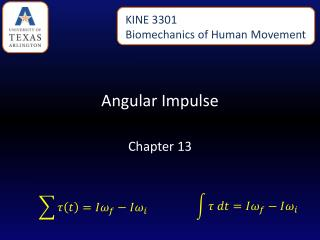Download PresentationAngular Impulse

Loading in 2 Seconds...

# Angular Impulse - PowerPoint PPT Presentation

KINE 3301 Biomechanics of Human Movement. Angular Impulse. Chapter 13. Linear and Angular Momentum. Recall from chapter 8 that linear momentum was defined as mass x velocity. Angular momentum is defined as moment of inertia x angular velocityI am the owner, or an agent authorized to act on behalf of the owner, of the copyrighted work described.
Download Presentation## Angular Impulse

An Image/Link below is provided (as is) to download presentation

Download Policy: Content on the Website is provided to you AS IS for your information and personal use and may not be sold / licensed / shared on other websites without getting consent from its author.While downloading, if for some reason you are not able to download a presentation, the publisher may have deleted the file from their server.

- - - - - - - - - - - - - - - - - - - - - - - - - - E N D - - - - - - - - - - - - - - - - - - - - - - - - - -
Presentation Transcript
1. KINE 3301 Biomechanics of Human Movement Angular Impulse Chapter 13

2. Linear and Angular Momentum • Recall from chapter 8 that linear momentum was defined as mass x velocity. • Angular momentum is defined as moment of inertia x angular velocity • Angular momentum is a vector , the direction of the vector is defined by the right hand rule. • The units for angular momentum are kg∙m2/s • Angular momentum describes the quantity of rotation that a body possesses.

3. Computing Angular Momentum The diver shown below is rotating with an angular velocity () of −4.2 r/s and a moment of inertia (I) of 5 kg∙m2, compute the magnitude of the divers angular momentum.

4. Angular Impulse – Angular Momentum Relationship • The angular impulse – angular momentum relationship is derived from Newton’s law of angular acceleration: Angular Impulse = change in angular momentum If you apply a torque over time (angular impulse), it changes the angular momentum of an object. An angular impulse is required to make an object spin or stop an object from spinning

5. An average torque of 3.72 N∙m is applied for a time of t = .9 s to the rigid bar shown below. Determine the final angular velocity of the bar.

6. A constant torque of 4.55 N∙m is applied to a rigid bar with a moment of inertia of 0.8 kg∙m2 and an initial angular velocity of 0.0 r/s, determine the final angular velocity. What was the angular impulse that was applied?

7. To compute the angular impulse for a non-constant torque the torque must be integrated with respect to time: After the torque is applied to the rigid bar the angular velocity increases to 5.27 r/s. If the rigid bar has a moment of inertia of 0.8 kg∙m2what was the final angular momentum? If the torque was applied for t = 0.9 s, what was the angular impulse?

8. Principle of Conservation of Angular Momentum When gravity is the only force acting on a system the angular momentum is constant.

9. During the force application phase the gymnast applies forces against the ground. The reaction forces cause torque about the gymnast’s center of mass. The net positive torque creates positive angular momentum. When the gymnast is in the air the net torque about the center of mass is zero and as a result angular momentum is constant. Is the moment of inertia constant? Is the angular velocity constant?

10. Use Excel to compute the angular impulse for each positive and negative torque phase for the torque curve shown below.

11. A Tale of Two Divers GuoJingjing Height 1.63 Mass 49 kg ICM = 5.6 kg∙m2 Shaquille O’Neal Height 2.16 m Mass 147.42 kg ICM = 29.58 kg∙m2 Each diver will perform a layout back 1 ½ rotation dive from a 10 m platform, they will be in the air for t = 1.68 s. Compute the following: 1.) angular velocity required to complete 1 ½ rotations (9.42 rad), 2.) angular momentum required, 3.) angular impulse required.